# Flooring Measurement Guide

## How to Measure a Square or Rectangular Room?

Measuring a square or rectangular room is very easy. All you need to do is measure the length and width of the room and multiply the numbers by one another. Length x Width = Area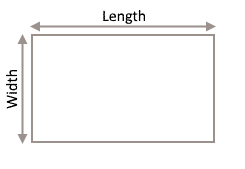### Square or Rectangular Room

Measuring a square or rectangular room is very easy. All you need to do is measure the length and width of the room and multiply the numbers by one another. Length x Width = Area
For example, if you room is a 4m x 3m, you have a 12m2 room.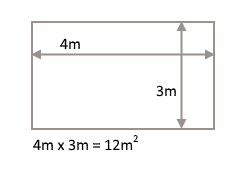Generally, you will want to add a 10% waste factor of the total size of your room. Just multiply your area by 1.1 and round upward. So, if your total area is 12m2, you will need to order enough flooring materials for 14m2.

Once you’ve got your rooms measured why not get a free estimation!

## How To Measure a Irregular Shaped Room?

If your room is not perfectly square or rectangular, such as an L-shape or trapezoidal shape, you will need to split up your room into two sections of squares or rectangular or even a triangle to calculate the area.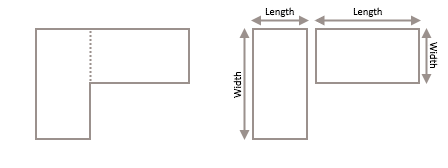Measure the length and width of each rectangle, calculate the total area of each and simply add the totals together to get the total size of your room. Don’t forget to add a 10% waste factor!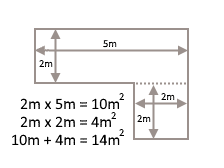For a room with trapezoidal shape or a diagonal corner, first you will need to measure the length and width of the rectangle and calculate the total area of the rectangle.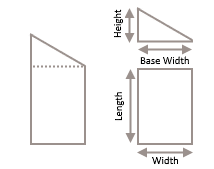Next, you will have to measure the triangle’s base width and height. Then, divide the base by 2 and multiply the number by height to get the total area of the triangle.
½ base x height = area

Finally, add the total area of the rectangle and triangle together. Remember to add the 10% waste factor!

Once you’ve got your rooms measured why not get a free estimation!

## How To Measure a Staircase?

Measuring a staircase may seem complicated but it will be a lot easier if you follow these 3 important steps:

### 1. Measure The Stair Width

Before you begin measuring, it is important to double check a few of your stairs in order to make sure they have the same width. Record the measurement.

### 2. Measure a Stair Tread and Riser

The stair tread is the horizontal part flat that you step onto. The stair riser is the vertical part of the stair on which the tread rests. First, measure the riser (height) and depth (tread) of one step. Add those numbers together and then multiply by the width. This will give you the measurements for one step.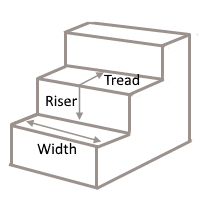### 3. The Number of The of The Stairs

Multiply your calculation from step 2 by the number of steps that you have.

## How To Measure a Winder Step?

Winders are the wedge rectangular steps which turn the stairs through 90 degrees. Usually there are 3 winder steps to consider. You’ll have to measure each step individually.

First, measure the width of the winder step at the widest point. Then measure height (riser) and depth of the tread of the winder step. Multiply these numbers by one another. Repeat the process for each winder step, then add the results to the total of the regular stairs.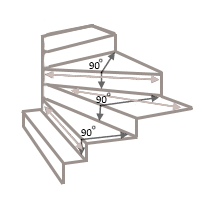Once you’ve got your stairs measured why not get a free estimation!